Previous: 3. Simulation - Theory Up: 3. Simulation - Theory Next: 3.1.1 One-Dimensional Point Response

# 3.1 Analytical Method

The analytical method is a purely empirical method. The distribution of the implanted particles is calculated by applying an analytical distribution function (point response function)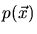to the simulation domain.

The point response function describes the distribution resulting from an implantation through a single point (ideally focused ion beam) of the surface of the simulation domain. Depending on the dimension of the simulation it is either a one-dimensional, two-dimensional or three-dimensional function fulfilling the condition that the integral over space is one.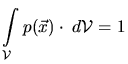(3.1)

The impurity concentration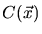at position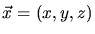is calculated summing up the contributions of all point response functions at all surface points of the simulation domain and multiplying by the implantation dose.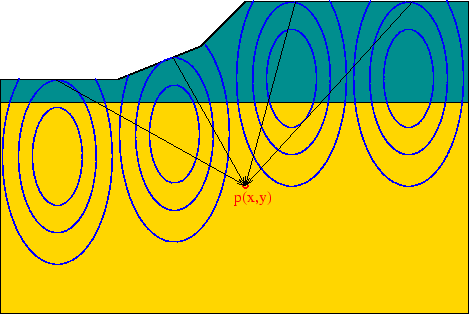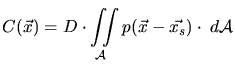(3.2)is a point at the surface of the simulation domain, and the integration is performed over the whole surface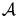.denotes the implantation dose. Due to the fact that point response functions are always defined in a coordinate system where the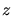axis is parallel to the ion beam the convolution in (3.2) is also performed in a coordinate system aligned with the ion beam.

SubsectionsPrevious: 3. Simulation - Theory Up: 3. Simulation - Theory Next: 3.1.1 One-Dimensional Point Response

A. Hoessiger: Simulation of Ion Implantation for ULSI Technology